# Earthwork Volume Using Simpson’s Rule

Step by step procedure to find out volume of earthwork using Simpson’s Rule

This article is about using Simpson’s rule (also known as Prismoidal Rule) to find out the quantity of earthwork by means of contour maps. The procedure is explained here with the help of an example. In the example here below, the map is divided in to 6 horizontal and 6 vertical grids each of 5m interval. The reduced levels are given at the intersections. Let us find out the volume of earthwork.Let us first note down the reduced levels in a tabular form.A three dimensional view will look like the following.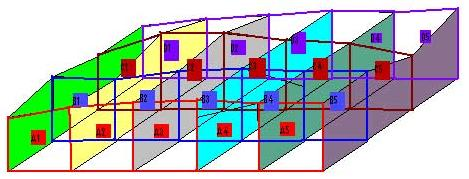Suppose the final level for filling is 24.1m. Then the required depth of filling at each point is as given in the table below.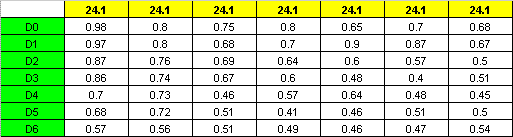The area on the side of each grid can be found out as follows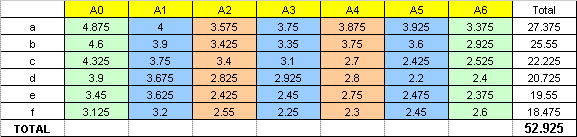Each side can be considered as a trapezoid. Hence the area of each side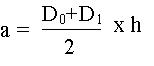eg: (0.98+0.97)/2 x 5 = 4.875

(0.80+0.80)/2 x 5 = 4.000

Now the volume according to Simpson’s rule is as follows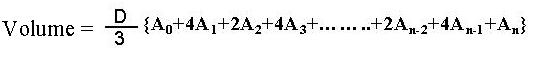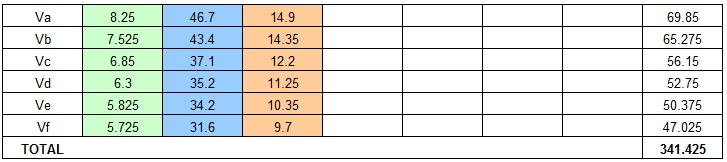eg: (4.875+3.375)=8.25

4 x (4+3.75+3.925)=46.7

2 x (3.575+3.875)=14.9

Total = 69.85

Total earth filling volume = 341.425 x (d/3)

=341.425 x (5/3) = 569.042 M3

Note: We express thanks to https://www.e-quantities.com/volumes.htm for bringing up an error in this page to our notice which now has been rectified. You may download the excel file detailing  volume calculation using Trapizoidal Rule here.

More Articles in Civil Engineering

Are Contractors Entitled for Variations in Lump Sum Contracts? – Read Article

What is Day Work in Construction Contracts? – Read Article

How to Calculate Earthwork Volume Using Simpson’s Rule? – Read Article

What is Contingency Allowance in a Construction Contract? – Read Article

What is Clause 14 programme? – Read Article

What is FIDIC? – Read Article

What is Prime Cost Sum in Construction Contracts? – Read Article

What is Provisional Sum in Construction Contracts? – Read Article

What is TOR Steel? – Read Article

## 2 Replies to “Earthwork Volume Using Simpson’s Rule”

1.Satish says:

Lovely

2.R Goddard says:

This is very good. The square base method gives a very similar result at 568.313 m3. Also tested it against the program at